How to Convert Time Format from 12-Hour Clock to 24 OR 24 to 12-Hour Clock in Excel

Sometimes we need to enter time into excel, and as we all know there are several formats to show the time. We often use 12-hour clock or 24-hour clock to show the time like 3:01:01PM or 15:01:01, one time displays in two different formats. So if we want to convert time format between them, how we can do? This article will show you the method to convert 12-hour clock to 24-hour clock in excel and vice versa.

Preset Time Format in Excel

If you just want to enter Time into excel by 12-hour clock or 24-hour clock format, you can preset your cell format to a proper one before entering the time.

Step1: Open a blank excel, select column A for example, right click, select ‘Format Cells…’.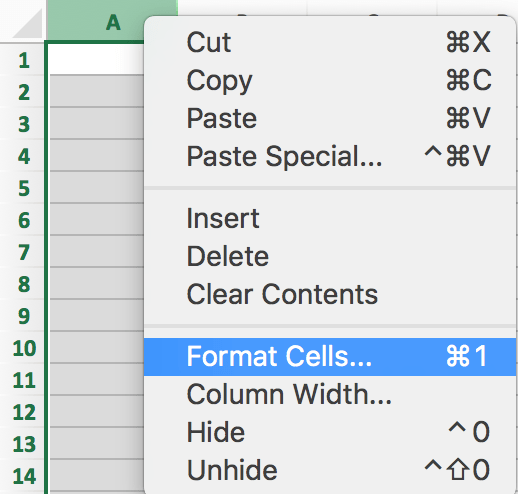Step 2: In Format Cells, under Number tab, select Time in Category list. Then all Types are loaded in the right. See below screenshot: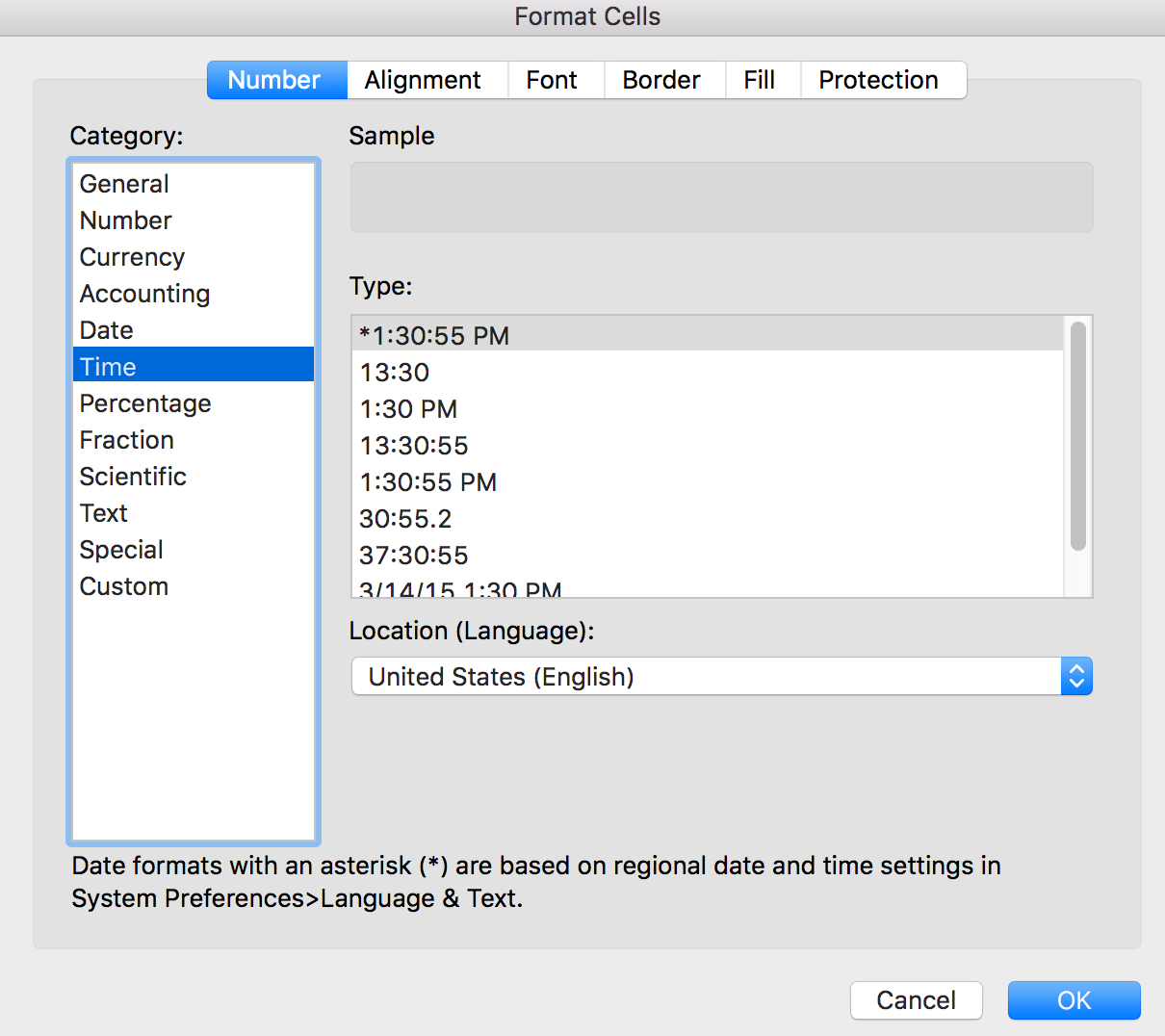Step 3: You can select the Type you expect to show the time when you entering time data into excel. For example, we select the first one 12-hour format to show the time, click OK on Format Cells to save the preset. Then in A1, we enter 13:55:55 directly. After inputting and clicking Enter, 1:55:55 PM is auto displayed in the cell.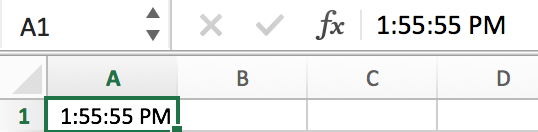If you already have a list of time in existing excel worksheet, how can we convert time format to expect time format? You can follow below steps to convert time format.

Convert from 12-Hour Clock to 24-Hour Clock

Step 1: Prepare a list of time in excel. See below.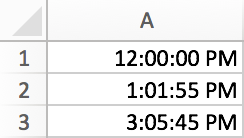Step 2: In column B->B1, enter formula ‘=TEXT(A1,”HH:MM:SS”)’, click Enter. Verify that PM is cleared properly.

Step 3: Copy B1 to B2 and B3 (or hold B1 and drag down to B3 directly). Time in A column is converted to 24-hour clock properly now.Convert from 24-Hour Clock to 12-Hour Clock

Step 1: Following above steps, a list of 24-hour format time is displayed in B column. Now in column C->C1, enter the formula ‘=TEXT(A1,”HH:MM:SS AM/PM”)’. Verify that 24-hour format is converted back to 12-hour format.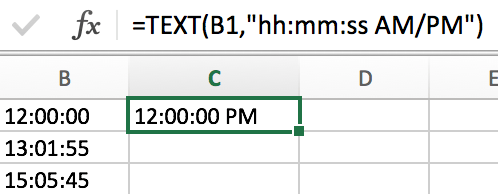Step 2: Use the same way to fill C2 and C3.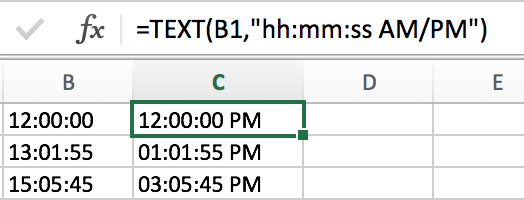Step 3: Be aware that the slash is “/”, if you entered “\” you may get below result improperly.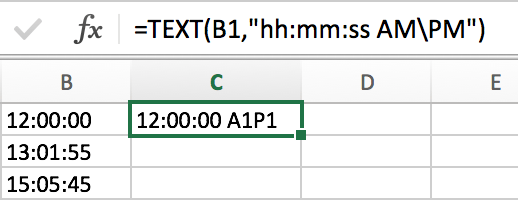Related Posts

Filter And Transpose Data From Horizontal To Vertical

This post will show you how to use Filter function and in combination with Transpose function to filter data from horizontal and transpose data as vertical in Microsoft Excel. You can refer to the below general formula based on TRANSPOSE ...

VLOOKUP Formula | Faster Trick with 2 VLOOKUPS

This post will guide you how to use 2 VLOOKUPS function to looking up data entries from a given range of cells in Microsoft Excel. VLOOKUP with 2 lookups can be faster than a single VLOOKUP in certain scenarios. The ...

Extract Unique Items From A List

This post will guide you how to extract unique itmes from a given list in Microsoft Excel. How to create a newly formula to get unique values from a range cells in Excel. The unique list of items is the ...

Extract substring In Excel

This post will guide you how to use Excel's MID function is a quick and easy way to extract pieces from your text. Use the Excel formula to extract a substring with MID. Note: If you want to extract just ...

How to Use 3D SUM Multiple Worksheets

To sum a range of numbers is straightforward for most Excel users, but do you know how to establish a 3D reference to total the same range of numerous sheets. In this post, I will present the steps for this ...

Extract all the matches with helper Column

With Excel's powerful functions IF, INDEX, and MATCH, we can find exactly what you're looking for with a few clicks of the mouse. This step-by-step tutorial will show how easy it is to extract data using these tools and more! ...

If Cell is This Value or That Value

IF function is frequently used in Excel worksheet to return you expect “true value” or “false value” based on the result of logical test. If you want to see if a cell is A or B, and if one of ...

If Value is Greater Than A Certain Value

IF function is frequently used in Excel worksheet to return you expect “true value” or “false value” based on the logical test result. If you want to see if a value in one cell is greater than a specific value, ...

If Cell is Not Blank

IF function is frequently used in Excel worksheet to return you expect “true value” or “false value” based on the result of created logical test. If you want to see if a cell is blank or not, and leave some ...

VBA Macro For VLOOKUP From Another Sheet

In the previous post, you should know that how to fix or remove the #N/A error when using VLOOKUP formula to lookup value from another sheet. And this post will show you how to use VBA code to vlookup data ...

Sidebar